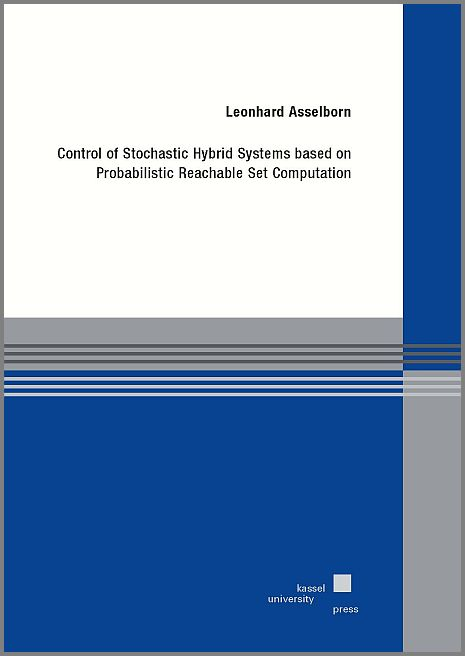Control of Stochastic Hybrid Systems based on Probabilistic Reachable Set Computation

Asselborn, Leonhard

kassel university press, ISBN: 978-3-7376-0580-9, 2018, 170 Pages

Zugl.: Kassel, Univ., Diss. 2018Content: This thesis proposes an algorithmic controller synthesis based on the computation of probabilistic reachable sets for stochastic hybrid systems. Hybrid systems consist in general of a composition of discrete and continuous valued dynamics, and are able to capture a wide range of physical phenomena. The stochasticity is considered in form of normally distributed initial continuous states and normally distributed disturbances, resulting in stochastic hybrid systems.

The reachable sets describe all states, which are reachable by a system for a given initialization of the system state, inputs, disturbances, and time horizon. For stochastic hybrid systems, these sets are probabilistic, since the system state and disturbance are random variables. This thesis introduces probabilistic reachable sets with a predefined confidence, which are used in an optimization based procedure for the determination of stabilizing control inputs. Besides the stabilizing property, the controlled dynamics also observes input constraints, as well as, so-called chance constraints for the continuous state.

The main contribution of this thesis is the formulation of an algorithmic control procedure for each considerd type of stochastic hybrid systems, where different discrete dynamics are considered. First, a control procedure for a deterministic system with bounded disturbances is introduced, and thereafter a probabilistic distribution of the system state and the disturbance is assumed. The formulation of probabilistic reachable sets with a predefined confidence is subsequently used in a control procedure for a stochastic hybrid system, in which the switch of the continuous dynamics is externally induced. Finally, the control procedure based on reachable set computation is extended to a type of stochastic hybrid systems with autonomously switching of the continuous dynamics.

Publication is available in following forms:

Full text (pdf-file, printable, Open Access - 2.08 MB)